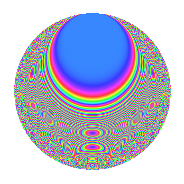# Properties

 Label 630.2.bqLevel 630 Weight 2 Character orbit bq Rep. character $$\chi_{630}(79,\cdot)$$ Character field $$\Q(\zeta_{6})$$ Dimension 96 Newform subspaces 1 Sturm bound 288 Trace bound 0

# Related objects

## Defining parameters

 Level: $$N$$ = $$630 = 2 \cdot 3^{2} \cdot 5 \cdot 7$$ Weight: $$k$$ = $$2$$ Character orbit: $$[\chi]$$ = 630.bq (of order $$6$$ and degree $$2$$) Character conductor: $$\operatorname{cond}(\chi)$$ = $$315$$ Character field: $$\Q(\zeta_{6})$$ Newform subspaces: $$1$$ Sturm bound: $$288$$ Trace bound: $$0$$

## Dimensions

The following table gives the dimensions of various subspaces of $$M_{2}(630, [\chi])$$.

Total New Old
Modular forms 304 96 208
Cusp forms 272 96 176
Eisenstein series 32 0 32

## Trace form

 $$96q + 48q^{4} - 4q^{6} + 2q^{9} + O(q^{10})$$ $$96q + 48q^{4} - 4q^{6} + 2q^{9} - 8q^{11} - 2q^{14} - 2q^{15} - 48q^{16} + 14q^{21} - 2q^{24} + 24q^{26} + 10q^{29} - 2q^{30} - 34q^{35} - 8q^{36} + 8q^{39} + 30q^{41} - 4q^{44} + 10q^{45} - 6q^{46} + 12q^{49} - 12q^{50} - 8q^{51} + 4q^{54} + 12q^{55} - 4q^{56} - 24q^{59} - 10q^{60} - 6q^{61} - 96q^{64} + 18q^{65} - 16q^{66} + 36q^{69} + 6q^{70} - 32q^{71} - 14q^{75} + 70q^{81} + 22q^{84} - 8q^{86} - 66q^{89} - 22q^{90} - 12q^{94} + 30q^{95} + 2q^{96} - 4q^{99} + O(q^{100})$$

## Decomposition of $$S_{2}^{\mathrm{new}}(630, [\chi])$$ into newform subspaces

Label Dim. $$A$$ Field CM Traces $q$-expansion
$$a_2$$ $$a_3$$ $$a_5$$ $$a_7$$
630.2.bq.a $$96$$ $$5.031$$ None $$0$$ $$0$$ $$0$$ $$0$$

## Decomposition of $$S_{2}^{\mathrm{old}}(630, [\chi])$$ into lower level spaces

$$S_{2}^{\mathrm{old}}(630, [\chi]) \cong$$ $$S_{2}^{\mathrm{new}}(315, [\chi])$$$$^{\oplus 2}$$

## Hecke Characteristic Polynomials

There are no characteristic polynomials of Hecke operators in the database